### Material estimation for M15 (1:2:4 mix ) grade concrete./Calculating the quantity of materials in 100 cu ft. & 1 cum. of M15 (1:2:4 ) grade concrete.

Let us consider 100 cu ft. of M15 grade concrete for the calculation purpose.

Here,

cement:sand: aggregates = 1:2:4 (a:b:c)

The wet volume of concrete = 100 cu ft.

The  dry volume of concrete

= 1.54 × 100 cu ft. = 154 cu ft.

1. Volume of cement required / 100 cu ft.

=[{ a ÷ (a + b + c)} × dry volume of concrete.]

= [{1÷ ( 1 + 2 + 4 )} × 154 cu ft.]

= [{ 1 ÷ 7 } × 154]

= [0.142857 × 154]

=  22 cu ft.

= 0.6229 cum.

(1cu ft = 0.028317 cum.)

As you know, 1 bag of cement = 0.03472 cum.

The number of cement bags required for 100 cu ft. of concrete

= [0.6229 ÷ 0.03472]

= 17.942 bags

The weight of cement

= [17.942 × 50 kgs.]

( 1 bag of cement = 50 kgs. )

= 897.1 kgs.

2. Volume of sand required / 100 cu ft of concrete

=( b ÷ (a + b + c)) × dry volume of concrete.

= (2 ÷ ( 1 + 2 +4 )) × 154 cu ft.

= ( 2 ÷ 7 ) × 154

= 0.2857 × 154

=  44 cu ft

3. Volume of aggregates required / 100 cu ft. of concrete

= [{ c ÷ (a + b + c)} × dry volume of concrete.]

= [{4÷ ( 1 + 2 +4 )} × 154 cu ft.]

= [{ 4 ÷ 7 }× 154]

= [0.5714 × 154]

=  88 cu ft.

4. Volume of water required.

Let us consider the w/c ratio for M15 grade concrete as 0.5.

i.e. wt. of cement/wt. of water = 0.5

So, the wt. of water

= [0.5 × wt. of cement]

= [0.5 × 897.1 kgs]

= 448.55 kgs.

= 448.55 liters

( 1kg = 1 liter.)

#### Alternate method:

1. Volume of cement ( from above) = 22 cu ft.

2. Volume of sand

= [volume of cement × b]

=   [22 cu ft. × 2]

= 44 cu ft.

3.  Volume of aggregates

= [volume of cement × c]

= [ 22 cu ft. × 4]

= 88 cu ft.

#### Quantity of materials in 1 cum of M15 (1:2:4) mix concrete:

As you know, 1 cum. = 35.3147 cu ft.

So, multiplying the above-derived quantity of cement / cum of concrete with (35.3147 cu ft  ÷ 100 cu ft. ), we will get,

1. Volume of cement in 1 cum. of M15 concrete

= [0.6229 cum. × (35.3147   ÷ 100  )]

= [0.6229 cum  × 0.353147]

= 0.22 cum.

The density of cement = 1440 kg/ cum.

The wt. of cement in kgs

= [0.22 cum. × 1440 kg/cum]

= 316.8 kgs

The number of cement bags

= [316.8 ÷ 50]

(1 bag of cement = 50kgs.)

= 6.336 bags.

2. Volume of sand in 1 cum of M15 concrete

= [volume of cement × b]

=  [ 0.22 cum. × 2]

= 0.44 cum.

= 15.538 cu ft.

( As 1CUM = 35.3147 cu ft.)

3.  Volume of aggregates in 1cum of M15 concrete

= [volume of cement × c]

=  [0.22 cum. × 4]

= 0.88 cum.

= 31.077 cu ft.

4. Volume of water in 1cum of M15 concrete

w/c ratio for M15 grade concrete is taken as 0.5.

wt. of cement/wt. of water = 0.5

So, the wt. of water

= [ 0.5 × wt. of cement ]

= [0.5 × 316.8 kgs.]

= 158.4 kgs.

= 158.4 liters

( 1kg = 1 liter.)

Now, let us put these material quantities in a table format for easy reference.

 Materials in 1CUM of M15 grade concrete. Sl. No. Item Unit In CUM. In cu ft. 1. Cement bags 6.336 bags - 2. Sand - 0.44 CUM 15.54 3. Aggregates - 0.88 CUM 31.08 4. Water litres 159 litres -

### Calculating the quantity of materials for the 100 sq.ft. of tile flooring.

Let us fix the tile of 2' × 2' size in the room of area 10 ft.× 10 ft.

Let us use cement mortar of 1:4 ratio having 40 mm. thickness to fix these tiles.

Calculation:

1.  The number of tiles required

= room area ÷ tile area

= [(10ft × 10 ft.)÷  (2 ft. × 2 ft.)]

= [100 sqft. ÷ 4 sqft.]

= 25 nos.

The wet volume of cement mortar

= room area × mortar thickness

= 100 sq. ft. × (40 × 0.00328084) ft.

( 1mm = 0.00328084 ft. )

= 100 sqft.× 0.13123 ft.

= 13.123 cu ft.

Dry volume of cement mortar

= 1.33 × 13.123 cu ft

= 17.453 cu ft.

= 0.4942 cum.

( 1cu ft = 0.0283168 cum. )

2. The volume of cement required

= dry volume of floor mortar ×  (ratio of cement  ÷ total ratio)

= 17.453 cu ft ×  ( 1 ÷ (1+4 ))

= 17.453 × 0.2

= 3.4906 cu ft.

= 0.09884 cum.

( 1cuft = 0.0283168 cum. )

As you know, the density of cement = 1440kg/cum.

So, the total quantity of cement required in kgs.

= 0.09884 cum. × 1440 kg/cum.

= 142.3296 kg

One bag of cement weighs 50kg

The number of cement bags required

= 142.329 ÷ 50

= 2.84 bags.

3. The volume of sand required

= dry volume of floor mortar ×  (ratio of sand  ÷ total ratio)

= 17.453 cu ft.×  ( 4 ÷ (1+4 ))

=  17.453 cu ft.× 0.8

= 13.9624 cu.ft.

= 0.3953 cum.

or

Volume of sand

= volume of cement × 4

= 0.09884 cum.× 4

= 0.3953cum.

or

Volume of sand

= total dry volume- the volume of cement

= 0.49421 cum - 0.09884 cum

= 0.3953 cum.

Density of dry sand = 1602 kg/cum.

Weight of sand required

= 0.3953 cum × 1602 kg/cum

=   633.387 kg.

### Calculating the quantity of materials in 1 cum. & 1cuft. of plastering mortar in different mix ratios.

Let us consider a 1 cum. of plastering mortar in a 1:4 ratio

The wet volume of the mortar = 1 cum.

Dry volume of the plaster mortar

= 1.33 × 1 cum.

= 1.33 cum.

The volume of cement required

= dry volume of plaster mortar ×  (ratio of cement  ÷ total ratio)

= 1.33 cum ×  ( 1 ÷ (1+4 ))

= 1.33 × 0.2

= 0.266 cum.

= 9.393 cu ft.

As you know, the density of cement = 1440kg/cum.

So, the total quantity of cement required in kgs.

= 0.266 × 1440

= 383.04 kg

One bag of cement weighs 50kg

The number of cement bags required

= 383.04 ÷ 50

= 7.66 bags.

The volume of sand required

= dry volume of plaster mortar ×  (ratio of sand  ÷ total ratio)

= 1.33 cum ×  ( 4 ÷ (1+4 ))

= 1.33 × 0.8

= 1.064 cum.

= 37.575 cu.ft.

( 1cu.m = 35.315 cu.ft. )

or

Volume of sand

= volume of cement × 4

= 0.266 cum.× 4

= 1.064 cum.

or

Volume of sand

= total dry volume- the volume of cement

= 1.33 cum - 0.266 cum

=1.064 cum.

Density of dry sand = 1602 kg/cum.

Weight of sand required

= 1.064 cum × 1602 kg/cum

= 1704.528 kg.

Quantity of water required

= weight of cement × w/c ratio

= 383.04kg × 0.6

= 229.82 kg i.e. 229.82 liter.

Note: Water is mixed depending upon the consistency required for the plastering. If you use plasticizers the requirement for water reduces.

Now we will consider plaster mortar in a 1:6 ratio.

The volume of cement required

= 1.33 cum ×  ( 1 ÷ (1+6 ))

= 1.33 × 0.14285

= 0.19 cum.

= 6.709 cu ft.

The total quantity of cement required in kgs.

= 0.19 × 1440

= 273.6 kg

One bag of cement weighs 50kg

The number of cement bags required

= 273.6 ÷ 50

= 5.472 bags.

Volume of sand

= volume of cement × 6

= 0.19 cum.× 6

= 1.14 cum.

Weight of the sand

= 1.14 cum. × 1602 kg /cum.

= 1826.28 kg.

To get the quantity of materials for 1 cu ft. of plastering mortar, you have to replace the unit cum. from cu ft.

sl.no.  particulars mix ratio cement     sand         cement
in cu ft.    in cu ft.     in bags
1          plaster               1: 4           0.266         1.064       0.217
mortar

2                                     1: 6             0.19           1.14          0.155

### Calculating the volume of excavation for a shed. / Estimation & costing of a shed ( part 1 ).

Let us consider a shed of dimension as shown below.

The plinth size of the shed is 10 ft. × 8 ft.

Let us build this shed with a 6" thick wall using solid concrete blocks.

So, the inner dimension of the shed will be 9 ft. × 7 ft. (after deducting wall thickness) & the centerline of the wall is 9.5 ft. × 7.5 ft. as shown below.

Now, we will excavate a trench of size 2 ft. depth & 1.5 ft. in width with 0.75 ft. on either side of the wall center.

Outer excavation length

= wall center length + (excavation width ÷ 2) × 2 nos.

= 9.5 ft. +( 1.5 ft ÷ 2) × 2

= 11 ft.

Outer excavation width

= wall center width + (excavation width ÷ 2) × 2 nos.

= 7.5 ft. +( 1.5 ft ÷ 2) × 2

= 9 ft.

Now, excavation for the long side of the wall

= (length × width × depth ) × 2 nos.

= (11 ft. × 1.5 ft. × 2 ft. ) × 2 nos.

= 66 cu.ft.

Excavation for the short side of the wall

= (9 ft - ( 1.5 ft × 2 sides) × 1.5 ft. × 2 ft. ) × 2nos.

=( 6 ft. × 1.5 ft. × 2 ft.) × 2 nos.

= 36 cu.ft.

Total volume of the excavation

= 66 cu.ft. + 36 cu.ft.

= 102 cu.ft.

Engineers way of doing estimation:

sl.no.   item           description    length     breadth   depth  nos.    qty.  unit
in ft.        in ft.       in ft.

1.   excavation   long side of wall     11          1.5         2.0       2      66   cu.ft.

2.                       short side of wall    6          1.5         2.0       2       36   cu.ft.

Total quantity =  102 cu.ft.

### Calculating the number of roofing sheets required for a shed. / Estimation & costing of a shed ( part 5 ).

Let us consider a shed of plinth size 8ft.× 10ft. as shown in the drawing.

Here, length of the shed L = 10ft., width W = 8 ft., height H1 = 9 ft., & H2 = 8ft.

Let us extend the roofing sheet length by 1ft. on the left & right sides of the shed, & by 1.75 ft. on the front & backside of the shed.

Now, we will calculate the area of the roof that should be covered by the roofing sheet.

Width side of the roofing sheet

Wr = [length of the shed+( roofing sheet extension × 2 sides)]

= [10 ft. + ( 1ft. × 2nos.)]

= 12 ft.

Length side of the roofing sheet

Lr = [sloping roof length of the shed + ( roofing sheet extension on back + front side)]

To calculate the sloping length of the shed, as shown above, we have to derive the hypotenuse of the triangle.

Sloping roof length

= √ ( 8ft × 8ft + 1ft. × 1ft.)

= √ 65 ft.

= 8.062 ft.

Roofing sheet length

Lr = 8.062 + (1.75ft. + 1.75ft.)

=  11.562 ft.

We will consider buying tata durashine roofing sheets for this shed.

The nearer standard length of the sheet for the calculated length (11.562) is 3660mm. or 12ft.

So we will go for 12 ft. length roofing sheets for this shed.

Now, the effective width of this sheet after overlapping is shown below.

Here, effective cover width We = 1015mm, total width Wt =  1072mm.

The number of roofing sheets required

= [width side of the roofing sheet for the shed ÷ effective width]

= [Wr ÷ We]

By converting the effective width unit into ft.

(1mm = 0.00328084ft.)

We = [1015mm × 0.00328084]

= 3.33 ft.

Number of roofing sheets

= [12ft ÷ 3.33 ft.]

= 3.6036 sheets

By rounding off the sheets, we will buy 4 numbers of 12 ft. length roofing sheets to cover this shed.

Now, what will be the maximum roofing width that you can cover using these 4 sheets at their given effective cover width?

i.e. effective cover width  × 4 nos. of roofing sheet

=   1015 mm.× 4 nos.

= 4060 mm.

= 13.3202 ft.

If you said yes, then you have done one of the 3 mistakes in roofing sheet calculations.

### How to calculate the quantity of materials required for cement plastering?/ Estimating the volume of cement & sand in plasterwork.

Let us consider a wall of  5m × 3m, that should be plastered internally.³

Let us plaster this brick wall in a 1: 4 ratio having 12mm. in thickness.

Here, length L = 5m., height H = 3m., thickness T = 12mm.

Area (A) of the wall that would be plastered

A   = [L × H]

= [5m × 3m]

A = 15 sq.m.

Volume of the plaster

= [surface area of wall × plaster thickness]

= [15 sq.m. × 0.012m.]

= 0.18 cum.

Let us take 20% extra material to fill the voids, joints, uneven surfaces & for wastage.

So, the actual wet volume of the plaster

= [0.18 +( 0.18 × 20 ÷ 100)]

= [0.18 + 0.036]

= 0.216 cum.

Dry volume of the plaster mortar

= [1.33 × 0.216]

= 0.2873 cum.

The volume of cement required

= [dry volume of plaster mortar ×  (ratio of cement  ÷ total ratio)]

= [0.2873 cum ×  ( 1 ÷ (1+4 ))]

= [0.2873 × 0.2]

= 0.05746 cum.

As you know, the density of cement = 1440kg/cum.

So, the total quantity of cement required in kgs.

= [0.05746 × 1440]

= 82.7424 kg

One bag of cement weighs 50kg

The number of cement bags required

= [82.7424 ÷ 50]

= 1.6548 bags.

The volume of sand required

= [dry volume of plaster mortar ×  (ratio of sand  ÷ total ratio)]

= [0.2873 cum ×  ( 4 ÷ (1+4 ))]

= [0.2873 × 0.8]

= 0.22984 cum.

= 8.116 cu.ft.

( 1cu.m = 35.315 cu.ft. )

or

Volume of sand

= [volume of cement × 4]

= [0.05746 cum.× 4]

= 0.22984 cum.

Quantity of water required

= [weight of cement × w/c ratio]

= [82.7424kg × 0.55]

= 45.51 kg i.e. 45.51 liter.

#### Now, let us find out the volume of materials required for plastering a 100 sq.m. of the brick wall.

The volume of cement required

= [(100sq.m ÷ 15 sq.m.) × 0.05746 cu.m.]

(As we have already calculated the material requirement for the 15 sq.m. wall surface.)

= [6.6667 × 0.05746]

= 0.383066 cum.

= 551.618 kg. of cement

= 11.03 bags of cement

(By similar procedure as we did it above.)

Volume of sand required

= [0.383066 × 4]

= 1.5346 cum.

= 54.196 cu.ft.

( 1cu.m. = 35.315 cu.ft.)

### 19 types of valves used in piping. / Different types of valves. / Types of plumbing valves.

The different types of valves with their images are given to gain the basic knowledge of them and their mechanism.

#### 1. Ball valve:

These valves contain hollow balls to control the flow of liquid. Ball valves are quarter-turn valves with their handles turning at 90degree in either direction to regulate the flow.

#### 2. Gate valve:

They contain gate-like barriers for the flow of water, that can be lifted up & down by turning the wheel-type handles. Gate valves are usually used for the complete flow of water without obstruction, rather than regulating the flow.

#### 3. Bibcock valve:

This is the most common type of valve, usually referred to as tap (valve). These valves are used in bathrooms, sinks, basins, etc. to control water flow.

#### 4.  Flapper valve:

You can observe such type of valves in the flush tank of the toilets, that controls the water flow. They contain flaps and rubber seals to open and close, according to the level of water in the flush tank.

#### 5. Plunger valve:

These types of valves are used when the pressure of the flow should be controlled precisely and safely. Plunger valves provide a symmetrical flow pattern due to the built-in cylinders and rings.

#### 6. Spool valve:

These types of valves are used in controlling the flow of hydraulic fluids. They are further classified as rotary or sliding type spool valves.

#### 7.  Needle valve:

Needle valves are used for the precise control of the flow pressure when the flow rate is in a lower quantity. They contain a needle-shaped plunger to regulate the flow.

#### 8. Pilot valve:

These types of valves are used for the limited supply of flow feed under higher pressure.

#### 9.  Clapper valve:

These valves allow the flow of feed in one direction. They have a mechanical device attached to the hinge for the opening and closing movement.

#### 10. Globe valve:

They contain a disc-shaped plug at the end of the shaft to control the flow. They operate on the linear motion system for the regulation of feed.

#### 11.  Pressure valve:

They are the safety valves used to control the maximum allowable pressure or to limit the pressure as per the safety norms. So the pressure valves are also said as relief valves.

#### 12. Choke valve:

They contain a solid cylinder within a hollow cylinder to control the flow. The inner cylinder blocks or opens to provide a steady flow of the feed.

#### 13.  Diaphragm valve:

In these types of valves, a flexible membrane known as a diaphragm is used for fluid control. They are throttle valves having bi-directional flow.

#### 14.  Pinch valve:

They contain rubber sleeves, that pinche when operated to obstruct the flow of materials. These types of valves are used in industrial productions like pharmaceuticals, food, beverage, cement factories, etc. to control the flow of granules.

#### 15.  Plug valve:

Plug valves contain tapered cylindrical or conical inner plug-like bodies to regulate the flow. They are quarter-turn valves having a 90-degree rotation of the handles.

#### 16.  Check valve:

They are non-return or one-way valves, that generally allows the fluid to pass in one direction. The check valves have two openings in the body, where one enables entry and the other provides exit of the entered fluid.

#### 17.  Butterfly valve:

These valves have a round disc body that turns in 90 degrees when operated to control the flow. Butterfly valves are used in handling the flow of slurries that contain suspended matters, as they provide free flow of the fluids without much obstruction.

#### 18.  Disc valve:

They are closure devices used in controlling fluids as well as airflow. In the diffuser system, you can find such valves for air control.  Disc-shaped body de-pressurizes the feed and helps in regulating the flow.

#### 19.  Foot valve:

The foot valve is a type of check valve, that allows unidirectional flow. They are installed at the end of the suction pipe for lifting water from the wells, tanks, using water pumps.

Plumbing pipes  👈 BACK

You can subscribe (free) below for the e-mail notification.

### Different types of fencing wires./ What are the different types of fencing mesh & wires?

Now, let us go through the different types of fencing wires & fencing wire mesh that we can use in fencing the boundaries.

They are,

#### 1. Barbed wire :

The fencing wires have barbs at regular intervals as shown in fig. Barbed wires are available in different types and designs, having varying thickness and spacing of the barbs and wires.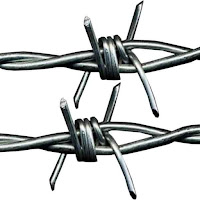Barbed wire.

#### 2. Razor wire:

Razor wire contains sharp blades instead of pointed arrow-like barbs, to provide extra security from the intruders. They are bundled into different forms and shapes as per their positioning in boundary fencing & applications.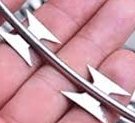Razor wire.

The wires are twisted, turned, and passed through one another to form a mesh. By removing or adding one twisted wire from the mesh, you can extend or break the mesh length as per your requirement. These twisted wires form a diamond shape when they are linked & installed in the boundary fencing.

They are available having a different wire diameter & with different types of protective coatings.Chain link mesh.

#### 4. Welded wire mesh:

They are manufactured by machine welding, forming different design patterns like square, rectangular, diamond, woven, etc. You have to use bolts, pins, hooks to anchor them for the non-metallic fence posts & you can weld them directly to the iron or MS posts to provide non-removable type boundaries.Welded mesh boundary.

### Based on the coatings:

#### 1. Vinyl coated fencing wires:Vinyl coating over the wire.

The polymer materials used for coating may be PVC or UPVC. They give robust protection against rusting from external weather conditions.PVC coated wire mesh.

#### 2.  Galvanized fencing wires:

The fencing wires were galvanized by providing a protective zinc coating over the surface. The most common way to coat is hot-dip galvanizing where the fencing wires are submerged in hot molten zinc.Zinc coated wire mesh bundle.

#### 3.  Galfan coated wires:

Zinc and aluminum alloy is coated over the fencing wires to provide excellent protection against rust. This is the new method of wire coating, that has more advantages when compared to the traditional zinc coatings. Some companies name them ZA-coated wires instead of Galfan wires.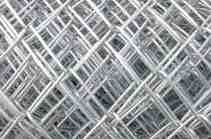Galfan coated wire bundle.

Have a good day.

### Different types of razor wires in fencing./ Various types of fencing razor wire.

The razor wires can be subdivided into the following four categories depending upon their application.

#### 1. Concertina razor wire:

Concertina razor wire is a cylindrical type of razor wire loop covering a wider space for extra security. These types of wire loops are used in forming the high and medium security fencing boundaries. Usually, you will find their usage in covering the airport grounds, military areas, chemical factories, etc.

They are twisted and curved to form double layers of protection, that create a high-level barrier for the trespassers.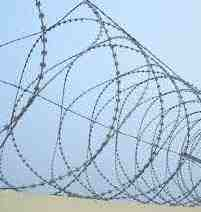concertina fencing.concertina wire loops.

#### 2. Flat wrap razor wire:

The spiral loops formed are flat, which is in line with the boundary fencing. They are usually installed as a top layer of fencing as shown in fig.

This loop shape makes it difficult for the intruders to jump or to pass through them.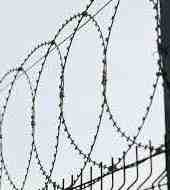flat wrap razor wire as a top boundary layer.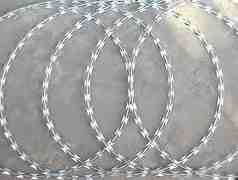flat wrap razor wire.

#### 3. Welded razor wire:

The razor wires are welded to form a diamond or square shape as shown in the fig. below. They can be used to replace the welded wire mesh fencing when extra security is needed. The razors in each mesh section intrude all types of animals from entering the premises.

These welded meshes are hooked, pinned, or bolted to the fence posts to create boundaries of the required height.diamond-shaped welded razor wire.square-shaped welded razor wire.

#### 4. Straight line razor wire:

They are the basic type of razor wires, where the wires are stretched in a single line similar to the barbed wires.

These types of wires are used over the boundaries as an extra security layer or for fencing the lands and buildings, where low or medium security is OK to protect them from trespassers.single-line razor wire.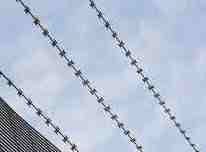straight line razor wire over boundaries.

Thank you for going through this article. Have a good day 😄.

### Different types of RCC fencing poles. / Various designs in RCC fencing poles.

RCC fencing poles are broadly classified under the following 3 sections.

A.  RCC fencing poles based on their design.

B. RCC fencing poles based on their thickness and shape. &

C. RCC fencing poles based on the size of the opening.

Now, we will observe the different types of RCC poles that come under each of these categories.

### A.  RCC fencing poles based on its design:

#### 1. Straight type RCC fencing pole:

They are a common type of fencing poles used for general purposes like fencing the plots, open sites, residential buildings, etc. The cross-sectional shape may be tapered, rectangular, or square, but they are straight in their length side without any angular bends.

#### 2. Bend type RCC fencing pole:

RCC poles are bend at the upper end usually at a 135-degree angle. These types of poles provide extra security as they make trespassing difficult to cross from above.

As you can see in the above fig., 3 nos. of barbed wires are fixed to the pole bend that gives a wider area at the top, making the boundary more secure.

#### 3. Y-type RCC fencing pole:

Y-type designed poles were used for the boundaries of high-security areas like factories, airports, military premises, etc.

As you can see in the above fig., razor wire fencing is used to fit in the Y-type pole, which helps in controlling the trespassers.

### B. RCC fencing poles based on their thickness and shape:

#### 1. Square-shaped RCC fencing pole:

These are the most common type of fencing poles widely used in covering residential plots, agricultural lands, marking boundaries of sites, etc. The ideal square shape is 4"× 4" having a varying length from 3ft. to 7ft.

#### 2. Rectangular-shaped RCC fencing pole:

Rectangular poles are good at taking tensions, as they are designed similar to the columns. They are good at taking the tension loads of the wires when the wider sides are placed parallel to the wire direction.

#### 3. Tapered-shaped RCC fencing pole:

Tapered-shaped poles are ideal for making the boundaries, where the fencing wire does not exert more tension over the poles. The shape of the pole narrows down at the top, on the two opposite faces of the RCC pole. Due to its tapering shape, they save the extra cost in the materials, and hence they are available at the lower price range when compared to other types of RCC poles.

### C. RCC fencing poles based on the size of the opening:

#### 1. Through type RCC fencing pole:

The openings are 1.25" to 1.5" in diameter so that you can pass the fencing wires directly through them. They are manufactured in higher grade RCC to withstand the pulling tension of the fencing wires.

#### 2. Pin type  RCC fencing pole:

The openings are smaller and you cannot pass the barbed wire through them. You have to use pins, hooks, or bolts with nuts to fix the barbed wire to these poles. These pin-type fence poles can be used for electric fencing or for the boundaries where the fencing wires are fixed for the external surface of the pole.